Courses

# Chapter Notes - Basic Geometrical Ideas Class 6 Notes | EduRev

## Class 6 : Chapter Notes - Basic Geometrical Ideas Class 6 Notes | EduRev

The document Chapter Notes - Basic Geometrical Ideas Class 6 Notes | EduRev is a part of the Class 6 Course Mathematics (Maths) Class 6.
All you need of Class 6 at this link: Class 6

Points, Lines and Curves:
The most practical branch of mathematics is geometry. The term 'geometry' is derived from the Greek word 'geometron'.

The most practical branch of mathematics is geometry. The term 'geometry' is derived from the Greek word 'geometron'. It means Earth's measurement. The fundamental elements of geometry are given below:

Point:
In geometry, dots are used to represent points. A point is used to represent any specific location or position. It neither has any size, nor dimensions such as length or breadth. A point can be denoted by a capital letter of the English alphabet. Points can be joined in different ways.

Line segment:
A line segment is defined as the shortest distance between two points. For example, if we mark any two points, M and N, on a sheet of paper, then the shortest way to join M to N is a line segment. It is denoted by Points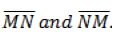are called the end points of the line segment.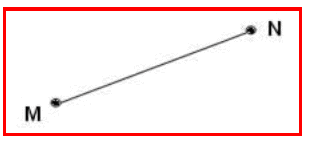Line:
A line is made up of an infinite number of points that extend indefinitely in either direction. For example, if a line segment from M to N is extended beyond M in one direction and beyond N in the other, then we get a line, MN. It is denoted by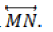A line can also be represented by small letters of the English alphabet.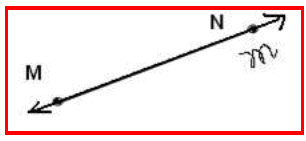Ray:
A ray is a portion of a line. It starts at one point and goes on endlessly in one direction. For example, if a line from M to N is extended endlessly in the direction of N, then we get a ray, MN. It is denoted byand can be read as ray MN.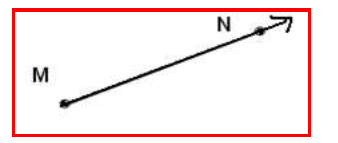Plane:
A plane is said to be a very thin flat surface that does not have any thickness, and is limitless. For example, this sheet is said to plane PQR. An infinite number of points can be contained within a plane.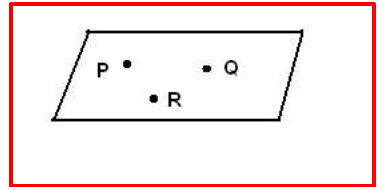Intersecting lines:
If two lines pass through a point, then we say that the two lines intersect at that point. Thus, if two lines have one point in common, then they are called intersecting lines. For example, two lines m1 and m2  pass though point P. These two lines are called intersecting lines.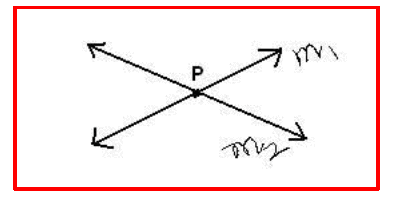Parallel lines or non- intersecting lines:
In a plane, if two lines have no point in common, then they are said to be parallel or non- intersecting lines.
Parallel lines never meet, cut or cross each other. In the figure, it can be observed that two lines m1 and m2  are parallel. We write . m1 || m2.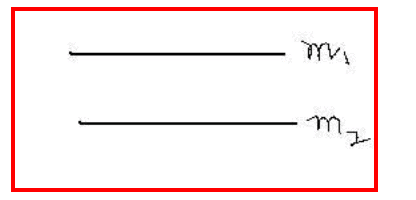Curves:
Curves can be defined as figures that flow smoothly without a break. A line is also a curve, and is called a straight curve. Curves that do not intersect themselves are called simple curves. The end points join to enclose an area. Such curves are called closed curves. For example, (i), (ii) and (iii) are simple curves, whereas (iv) and (v) are closed curves.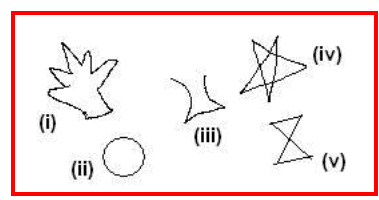For a closed curve, we can identify three regions:

• The interior of the curve. Here, point P is in the interior of the circle.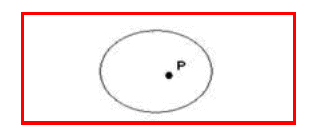•  Boundary of the curve. Here, point P is on the circle.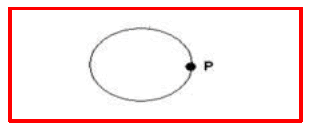•  Exterior of the curve. Here, point P is in the exterior of the circle.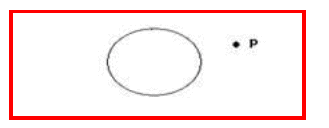Angles, Polygons and Circles: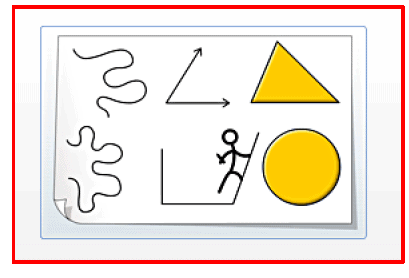An unknown quantity can be represented by a variable
Simple open curves made up of only two lines are called angles. Simple closed curves made up of more than two lines are called polygons. A circle is a simple closed curve formed by a point moving at the same distance from a fixed point.
Curves:
Figures that can be drawn without lifting the pencil from the paper and without the use of a ruler are called curves. Curves that do not cross themselves are called 'simple curves'. In the diagram here, (i), (ii), (iv) and (v) are simple curves, while (iii) is not a simple curve. Also, (i), (iii), (iv) are examples of closed curves, while (ii) and (v) are examples of open curves.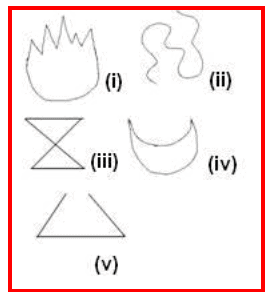An 'angle' is made up of two rays having a common end point. The two rays forming the angle are called 'sides' of the angle, and the common end point is called the 'vertex' of the angle. Simple closed curves made up of only line segments are called polygons. A polygon made up of three line segments is called a triangle.

For example,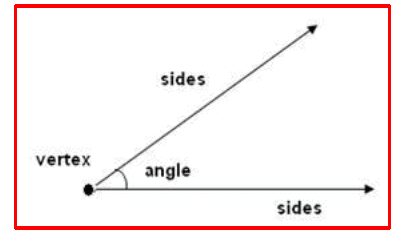The triangle in the diagram is called ΔABC . Here, points A, B and C are the vertices.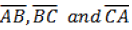are the sides, and ∠ ABC, ∠BCA and ∠CAB , and are the angles of the triangle.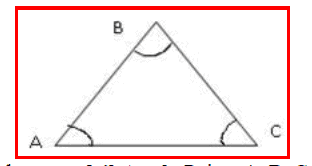A four-sided polygon is said to be a quadrilateral. Points A, B, C and D are the vertices.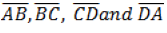are the sides, and ∠A, ∠B, ∠C and ∠D, and are the angles of quadrilateral ABCD. A quadrilateral has, in all, four pairs of adjacent sides. They are AB and BC, BC and CD, CD and DA, and DA and AB.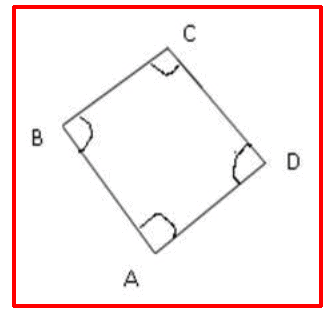Also, it has two pairs of opposite sides. They are AB and DC, and BC and AD. Angles A and B, angles B and C, angles C and D, and angles D and A are said to be adjacent angles. Angles A and C, and angles B and D are said to be pairs of opposite angles.

A circle is formed by a point moving at the same distance from a fixed point. A circle is also a simple closed curve; however, it does not have any sides or angles.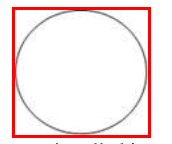The line that forms the boundary of a circle is called its circumference. The part enclosed by the circumference of a circle is called the interior of the circle. The part left outside the circle is said to be the exterior of the circle. Some points may lie on the circumference of the circle.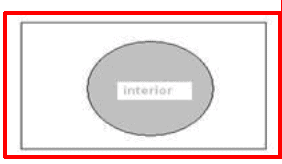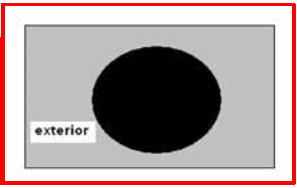An arc is a part of the circumference of a circle. A chord is a line segment joining two points that lie on a circle. The part of a circle that is enclosed by a chord and an arc is called a segment of the circle. A chord passing through the centre of the circle is called its diameter. A diameter is the longest chord of a circle. A diameter of a circle divides it into two halves. Each half is called a semi-circle. A line segment that joins the centre of the circle and a point on the circumference is called the radius of the circle. The radius of a circle is half of the diameter. The part of a circle enclosed by two radii and an arc is called a sector.

Offer running on EduRev: Apply code STAYHOME200 to get INR 200 off on our premium plan EduRev Infinity!

## Mathematics (Maths) Class 6

191 videos|220 docs|43 tests

,

,

,

,

,

,

,

,

,

,

,

,

,

,

,

,

,

,

,

,

,

;